## 10 Common Statistical Models You Should Know

#### Quantitative Model

Here presents the 10 most commonly used statistical models/algorithms every Quants and AI developers should know.

• Linear Regression
• Logistic Regression
• Decision Tree
• K-Nearest Neighbour
• K-Means Clustering
• Support Vector Machine
• Principal Component Analysis
• Naive Bayes
• Artificial Neural Network### 1. Linear Regression

Linear regression is a process for modelling the linear relationship between a scalar response (dependent variable) and one or more explanatory variables (independent variables).

In simple linear regression, we try to find the best fitting line that describes the relationship between one independent variable and one dependent variable. The independent variable is used to predict the dependent variable, and the line of best fit is used to estimate the value of the dependent variable based on the value of the independent variable.

To estimate the line of best fit, we can use the least squares method, which minimizes the sum of the squared differences between the predicted values and the actual values.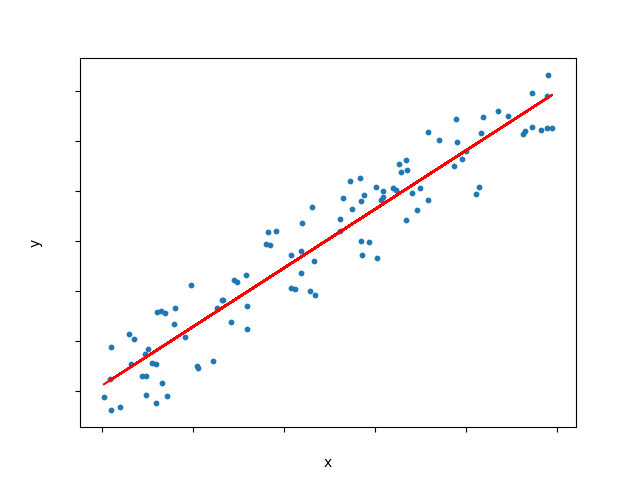Pros
Cons
• Easy to implement and fast to train
• Performs well when dataset is linearly separable
• Overfitting can be avoid by variable transformation
• Dataset is assumed to be independent which is rare in real life
• Sensitive to outliners
• Prone to noise and overfitting

### 2. Logistic Regression

Logistic regression is used to model binary outcomes (i.e. only two possible values, usually 0 or 1) with one or more independent variables. It is based on the logistic function, which transforms a linear combination of the predictor variables into a probability value between 0 and 1. The logistic regression algorithm estimates the coefficients of the predictor variables that maximize the likelihood of the observed data.

Logistic regression can also be extended to handle more complex scenarios, such as multinomial logistic regression for multiple categories or ordinal logistic regression for ordered categories.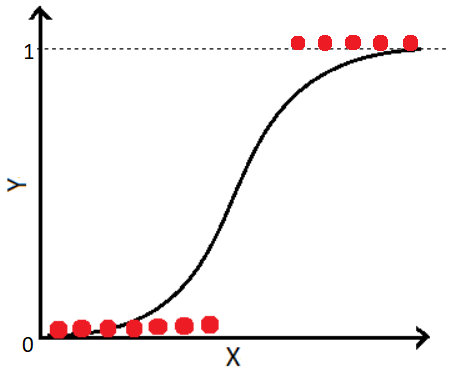Pros
Cons
• Easy to implement and fast to train
• Less prone to over-fitting but it can overfit in high dimensional datasets
• Efficient when the dataset has features that are linearly separable
• Dataset is assumed to be independent which is rare in real life
• Can only be used to predict discrete functions
• Should not be used when the number of observations are lesser than the number of features

### 3. Decision Tree

A decision tree model is used to make decisions by visually representing a sequence of decisions and their possible consequences. It is a type of supervised learning algorithm that can be used for both classification and regression problems. Decision trees are constructed by recursively splitting the data into smaller subsets based on a set of decision rules, with each split being made to maximize the information gain or minimize the impurity of the resulting subsets.

Decision trees are popular in machine learning because they are easy to understand and interpret, and they can handle both numerical and categorical data. They can be used for a variety of applications, such as predicting customer churn, diagnosing medical conditions, and identifying credit risk. Additionally, decision trees can be used in an ensemble of models, such as random forests and gradient boosting, to improve their predictive power.Pros
Cons
• Easy to visualize and explain
• Can solve non-linear problem
• Can work on high-dimensional data with excellent accuracy
• Calculations can get very complicated
• A small change in the data can lead to a large change in the struture of the optimal tree
• Prone to overfitting

### 4. K-Nearest Neighbour (KNN)

K-Nearest Neighbors (KNN) is a non-parametric machine learning algorithm that can be used for both classification and regression problems. It is based on the assumption that similar things are near to each other. The algorithm works by finding the K nearest data points in the training set to the new data point, and then assigning the classification or regression value based on the majority vote or average of the K nearest neighbors.

KNN is a simple and intuitive algorithm that does not make any assumptions about the underlying data distribution. It can handle both numerical and categorical data and can be used for both binary and multi-class classification problems. However, the algorithm can be computationally expensive as the number of training data points increases, and it may not work well with high-dimensional data.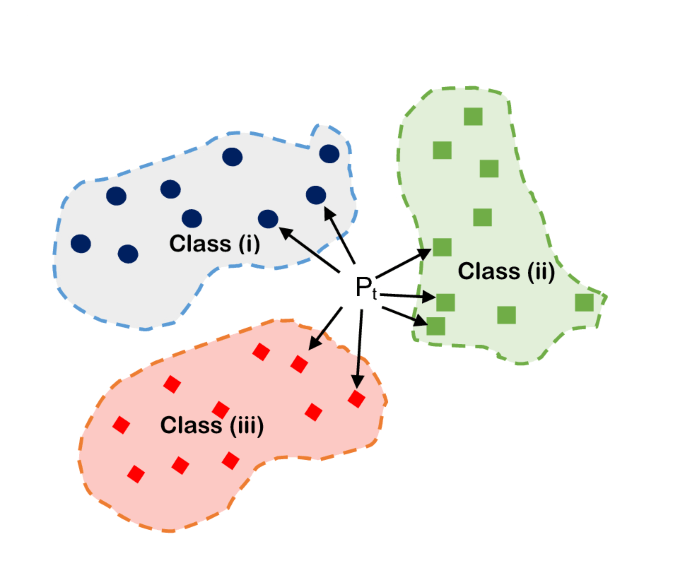Pros
Cons
• Can make prediction without training
• Can be used for both classification and regression
• Efficient in calculation
• Does not work well with large dataset
• Sensitive to noise, missing values and outliners
• Need feature scaling
• Choose the correct K value

### 5. K-Means Clustering

K-Means Clustering is an unsupervised machine learning algorithm used to partition a dataset into a set of K clusters based on the similarity of the data points. The algorithm works by iteratively assigning data points to the nearest centroid and updating the centroids until convergence.

The K in K-Means Clustering represents the number of clusters that the algorithm should form. The value of K is usually determined by the user, based on some prior knowledge about the dataset or by running the algorithm multiple times with different values of K.

K-Means Clustering is widely used in various applications such as customer segmentation, anomaly detection, and image segmentation. It can handle large datasets and is computationally efficient, making it ideal for clustering large datasets. However, the resulting clusters may depend on the initial positions of the centroids, and the algorithm may not work well if the clusters have non-spherical shapes or if the data points are not well-separated.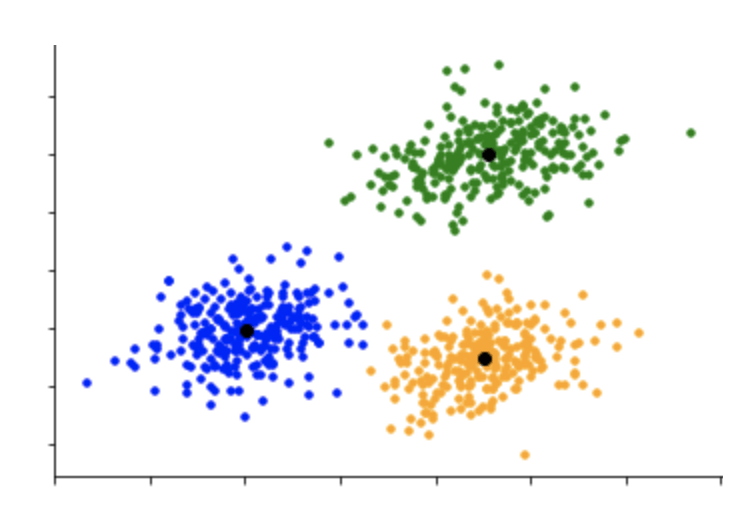Pros
Cons
• Simple to implement
• Can scale to large datasets
• Guarantees convergence
• Easily adopts to new inputs
• Generalizes to clusters of different shapes and sizes
• Dependent on initial values
• Sensitive to outliners
• Scalability decreases when dimension increases
• Manual choice of K

### 6. Support Vector Machine (SVM)

Support Vector Machine (SVM) is a supervised machine learning algorithm used for both classification and regression problems. The algorithm works by finding the best hyperplane that separates the data into different classes, where the hyperplane is defined as the linear decision boundary that maximizes the margin between the classes.

SVM is particularly useful when dealing with non-linearly separable data by using a kernel function to transform the input space into a higher-dimensional feature space, where the data may become linearly separable. The kernel function allows SVM to handle complex data distributions without explicitly defining the mapping function.

SVM is widely used in a variety of applications such as image classification, text classification, and gene expression analysis. It is a powerful algorithm that can handle high-dimensional data and has a good generalization performance. However, SVM may be sensitive to the choice of kernel function and the tuning of hyperparameters, and it may not perform well with large datasets.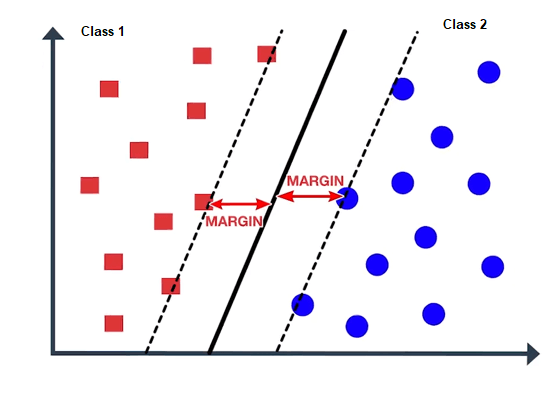Pros
Cons
• Good at high dimensional data
• Can work on small dataset
• Can solve non-linear problems
• Inefficient on large dataset
• Requires choosing the right kernal

### 7. Principal Component Analysis (PCA)

Principal Component Analysis (PCA) is a mathematical technique used to reduce the dimensionality of a dataset while retaining as much of the variability in the data as possible. The technique works by identifying the directions in the data that explain the most variation and projecting the data onto those directions.

PCA aims to find a set of new variables, called principal components, that are linear combinations of the original variables and capture the most important patterns in the data. The first principal component captures the largest amount of variance in the data, followed by the second principal component, and so on.

PCA is widely used in various fields such as image processing, bioinformatics, and finance. It can be used for exploratory data analysis, data visualization, and data compression. Additionally, PCA can be used as a pre-processing step for other machine learning algorithms to reduce the dimensionality of the input space and improve the performance of the model.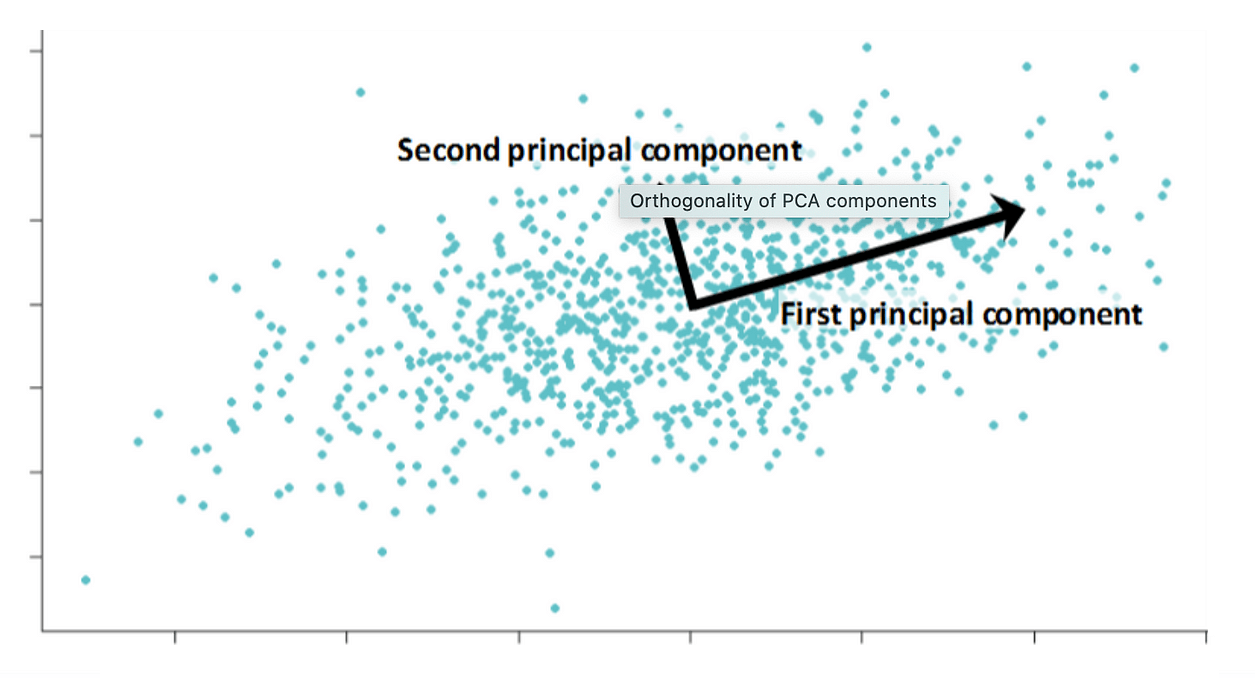Pros
Cons
• Reduce correlated features
• Improve performance
• Reduce overfitting
• Principal components are less interpretable
• Information loss
• Must standardize data before implement PCA

### 8. Naive Bayes

Naive Bayes is a simple yet effective probabilistic machine learning algorithm used for classification and prediction problems. The algorithm is based on Bayes' theorem, which states that the probability of a hypothesis is proportional to the conditional probability of the evidence given that hypothesis.

Naive Bayes assumes that the features of the data are conditionally independent given the class, which means that the presence or absence of one feature does not affect the probability of the other features. This assumption simplifies the computation of the conditional probabilities and allows for fast and efficient training and prediction.

Naive Bayes is widely used in many applications such as spam filtering, sentiment analysis, and document classification. It can handle both binary and multi-class classification problems and works well with high-dimensional and sparse data. Additionally, Naive Bayes requires relatively little training data and can be trained in real-time.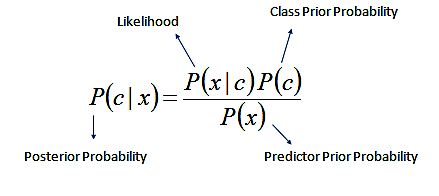Pros
Cons
• Short training time
• Suitable for categorical inputs
• Easy to implement
• Dataset is assumed to be independent which is rare in real life
• Estimation for zero frequency can be inaccurate

### 9. Artificial Neural Network (ANN)

Artificial Neural Networks (ANNs) are a set of machine learning algorithms inspired by the structure and function of biological neural networks in the human brain. ANNs consist of layers of interconnected nodes, called neurons, that process and transmit information. ANNs can be classified into different types, including feedforward networks, convolutional neural networks, and recurrent neural networks. Each type has its own unique architecture and is suited for different types of data and applications.

ANNs are capable of learning complex patterns and relationships between inputs and outputs, making them useful for a wide range of applications, such as image recognition, speech recognition, and natural language processing. The network learns by adjusting the strengths of the connections between neurons, based on the patterns in the training data.

ANNs require large amounts of training data and significant computational resources for training and inference. However, they are widely used in industry and academia due to their high accuracy and ability to learn complex patterns and relationships in the data.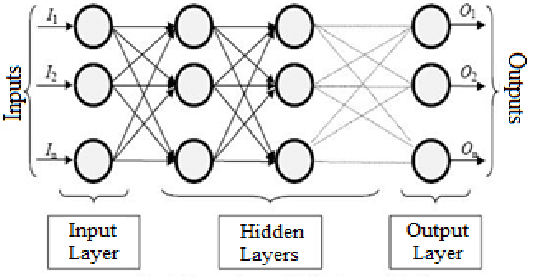Pros
Cons
• Have fault tolerance
• Able to learn and model non-linear and complex relationship
• Can generalize to unseen data
• Black box and hard to explain results
• Long training time
• Not guaranteed convergence
• Hardware dependence
• Requires user to translate the problem

Adaptive Boosting (AdaBoost) is a popular machine learning algorithm that is used for classification and regression problems. The algorithm works by combining multiple weak learners, such as decision trees or neural networks, to create a strong learner that can accurately classify data.

AdaBoost works by iteratively training weak learners on the data and assigning higher weights to the misclassified samples in each iteration. The algorithm then combines the weak learners by assigning weights to their predictions based on their performance. The final prediction is made by the weighted sum of the predictions of the weak learners.

AdaBoost is widely used in various applications such as face detection, text classification, and fraud detection. It is particularly useful when dealing with imbalanced datasets, where the number of samples in one class is much larger than the number of samples in the other class.

Overall, AdaBoost is a fast and efficient algorithm that can achieve high accuracy and is robust to noise in the data. It is often used in combination with other algorithms to improve the overall performance of the system.Pros
Cons
• Relatively robust to overfitting
• High accuracy
• Easy to understand and visualize
• Sensitive to noise data and outliners
• Not optimized for speed

### Summary

These summarize the 10 most widely used statistical tools which can handle many real world data modelling problems.

In my next articles, I will discuss how to implement these models for algorithmic trading. If you find my articles inspiring, like this post and follow me here to receive my latest updates.

Enter my promote code "AjpQDMOSmzG2" for any purchase on ALGOGENE, you will automatically get 5% discount.

very useful, thank you!